﻿ 激光振动测试仪在振动测试教学中的应用

# 激光振动测试仪在振动测试教学中的应用Application of Laser Vibration Instrument in Vibration Test Teaching

Abstract: The application of a novel laser vibration tester in vibration monitoring experimental teaching is discussed. Firstly, the main characteristics and use occasions of the instrument are analyzed. Secondly, the experimental bench and experimental steps are designed. Thirdly, the different parameters under the condition of the test results are compared. Then, the time domain curve and the frequency domain characteristic curve of the vibrating body are obtained. Finally, the factors affecting the test results and the precautions are discussed, which lays a foundation for the application of laser vibration tester in engineering practice.

1. 引言

2. 仪器工作原理和基本结构

2.1. 激光工作原理(a) 从低能级跃迁到高能级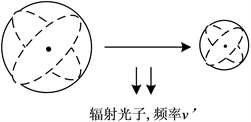(b) 从高能级跃迁到低能级

Figure 1. Electron transition in different energy level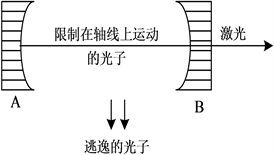Figure 2. Resonant cavity of laser derivation

$d=m\frac{\lambda }{2},m\in Z$，因此，激光的频率为： $\upsilon =\frac{mc}{2d}$。c为光速。因此，理论上可以得到不同频率的激光，其大小是 $\frac{c}{2d}$ 的整数倍。如果光源发射的激光具有相同的相位，则形成相干光。激光的相干性与频率数成

2.2. 基于多普勒效应的激光测振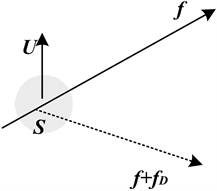Figure 3. Doppler phenomenon of acoustic source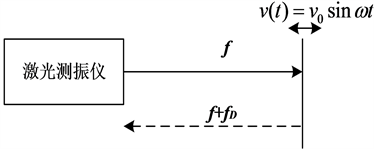Figure 4. Doppler effect of vibration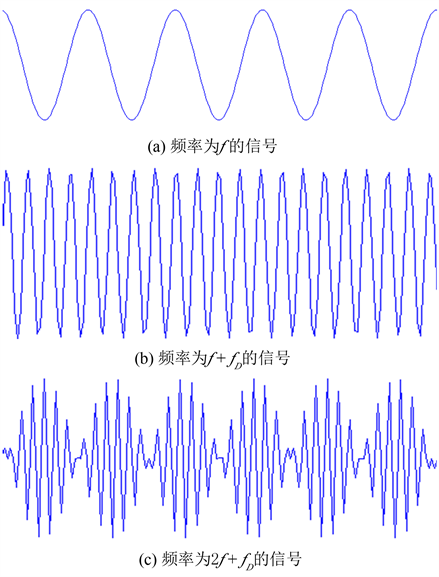Figure 5. Process of signal modulationFigure 6. Diagram of laser signal of modulation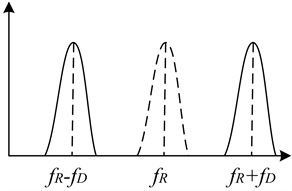Figure 7. Demodulation to extract Doppler frequency

3. 试验台架Figure 8. Laser vibration test instrument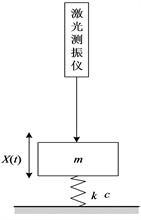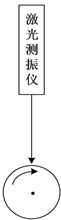(a) 非旋转部件的振动测试 (b) 旋转部件的振动测试

Figure 9. The application of the PDV100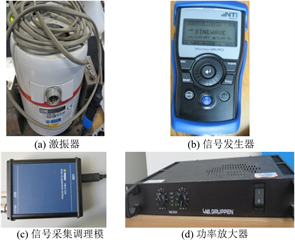Figure 10. The auxillary instruments in the experiment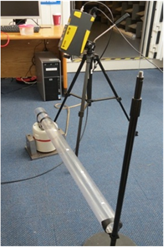Figure 11. The rig of the pipe vibrationTable 2. Laser vibrometer parameter setupTable 3. Parameter of pipeline system

4. 实验步骤

a) 将激光测试仪安装在三脚支架上，连接仪器和调理模块，通过数据线将调理模块与电脑连接；检查检定证书在有效期内；

b) 接通仪器电源，打开测试软件；

c) 调整激光挡板，并将激光聚焦在被测物体表面的反光带上；

d) 设置仪器的参数，主要包括滤波器的设置(低通滤波和高通滤波两种)和测试量程(20 mm/s, 100 mm/s, 500 mm/s)；

e) 调整激光测试仪和被测对象之间的距离，根据显示屏上信号区的尺度判断距离选择是否合适，距离合适则信号区的尺度大；

f) 调整信号发生器的频率，驱动振动台工作；调整功率放大器，改变得振动台的振动幅值；

g) 通过振动测试软件，观察时域波形和频域波形；调整采样频率和采样时间，再次观察振动信号曲线，采集数据并保存。

5. 实验结果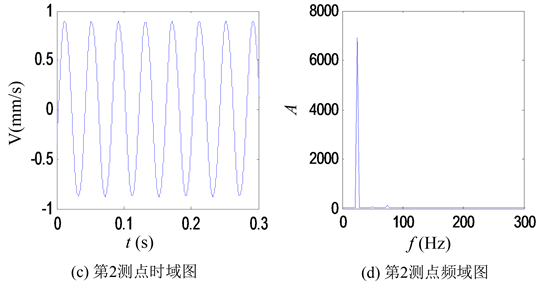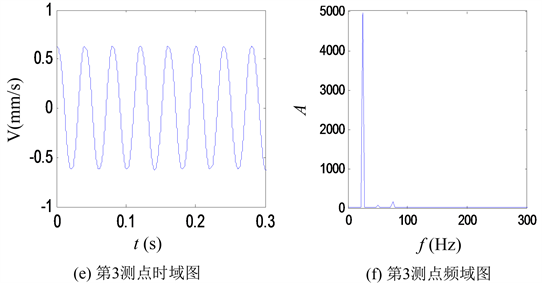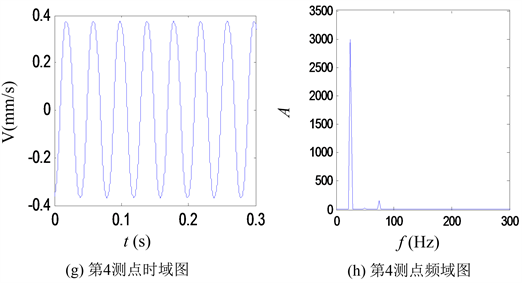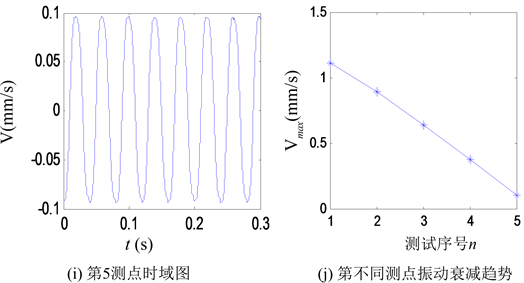Figure 12. Vibration response analysis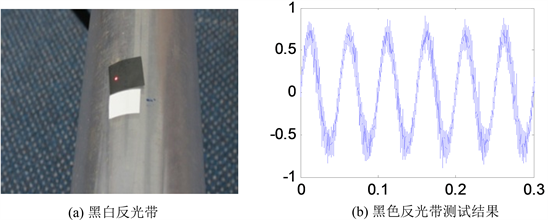Figure 13. Influence of reflective tape

6. 结语

NOTES

*通讯作者。

 肖涵, 吕勇, 王涛. 齿轮振动信号的去趋势波动分析及其在故障分类中的应用[J]. 振动工程学报, 2015(2): 331-336.

 孙福兆. 机械传动设备齿轮箱振动频率过高检测方法[J]. 计算机测量与控制, 2014(1): 66-84.

 韩春杨, 姚国凤, 赵建. 柴油机振动信号盲分离组合算法[J]. 振动与冲击, 2014(6): 44-52.

 孙斌, 梁超, 尚达. 基于递归复杂网络的滚动轴承故障诊断[J]. 振动、测试与诊断, 2015(3): 578-584.

 马宏伟, 王增强, 陶美华. 轴承振动信号特征提取及故障诊断研究[J]. 煤矿机械, 2014, 35(7): 278-280.

 周国强, 甘少炜, 雷伟, 等. 应变测量在轴系扭转振动测试中的应用[J]. 船海工程, 2014(2): 165-172.

 桑波, 赵宏, 谭玉山. 激光多普勒扭转振动测试技术的研究[J]. 中国激光, 2003, 30(8): 743-746.

 骆德渊, 任雅萍. 双频激光多谱勒扭转振动测试仪的研究[J]. 激光与红外, 1999(1): 19-20.

Top